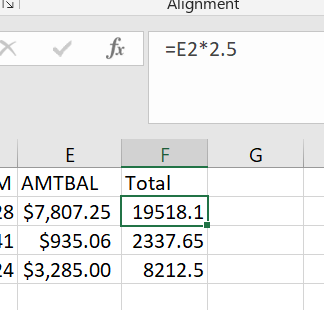# StudioX - Calculate using spreadsheet issue

Using StudioX. I have a spreadsheet with 35 rows and 7 columns. Has headers. I have Amount in one column. I use Use Excel → For each row to read the rows. I have a last column called ‘Total’. For this column, I take the value of each row from the Amount column and multiply it with 2.5 and write it corresponding ‘Total’ cell. While doing this it shows can error ‘Cannot convert Excel Value into Integer’ in Write Cell. I do this in Write Cell ‘What to Write’ (CInt(CurrentRow.ByField(“Amount”))*2.5 but I get an error. Any idea why am I getting an error ?

@Prinal_C
Instead of CInt try CDbl because you are multipling by 2.5 so the “Total” column value will be in double ie 35*2.5 = 87.5

@Karuna
This does not work either. I still see this error in Write Cell

Cannot convert Excel Value to System.Double

Try to change the "Total "variable type to “System.Double”

well, ‘Total’ is the column that needs to be filled up / written after calculation. This means in Write Cell “Where to Write”, I need to change to System.Double. How will this work ? It will show an error that it cannot convert into UiPath.Excel.ReadWriteCellRef.

@Prinal_C …could you please show us the sample of your spreadsheet? If you sensitive data you can mask that with dummy one …

@prasath17 screenshot attached. I’m trying this in StudioX.

@Prinal_C
Try this CalculatingAmount.zip (19.4 KB)

CalculatingAmount (2).zip (19.4 KB)

@Karuna … Thank you ! This works in Studio very well. However, I’m looking for a solution in StudioX only. Can somebody pls assist ?

Try to implement the same with StudioX Activities.

It’s not working in StudioX right from the beginning … that’s why the question was asked.

@Prinal_C - Is this what you are trying to achieve?@Prinal_C - Here you go…

Idea I have used here is : First trying to find the last row of any columns…which gives me the output: 4

1. Using this value, i can set my range for the column F…

Say : F2: F4

Excel.Sheet(“Sheet1”).Range(“F2:F” + Saved.Values(Of Int32)(“Last Data Row”).ToString)

Here: Saved.Values(Of Int32)(“Last Data Row”).ToString) ==> has the value of 4…

1. In the what to write = I am giving the formula as E2*2.5

That’s it here is my output2 Likes

@prasath17 … YES! This is what I was expecting as an outcome. Thank you again !
In Where to Write of Write Cell activity I also tried doing like this:
Excel.Sheet(“Sheet1”).Range(string.Format(“F{0}:F{1}”, Saved.Values(Of Int32)(“FirstRow”).ToString, Saved.Values(Of Int32)(“LastRow”).ToString))

1 Like

Awesome…

Glad to know…it is working for you…

1 Like

This topic was automatically closed 3 days after the last reply. New replies are no longer allowed.